# Redshift of Spectral lines in the Sun's Chromosphere.

### by Paul Marmet (1932-2005)

This is an adapted version of the paper in IEEE Transactions on Plasma Science, Vol. 17 No: 2, April 1989

( Last checked 2018/01/16 - The estate of Paul Marmet )
Eine deutsche Übersetzung dieses Artikels finden Sie hier.
This paper was written when the author was at:
L'institute Herzberg d'Astrophysique,
Conseil National de Recherches,
Ottawa, Ontario K1A 0R6

Abstract
An unexplained center-to-limb variation of solar wavelength has been known for 75 years.  Many theories have been developed in order to explain its origin.  Although recent studies reveal a large amount of new information on the solar chromosphere, such as asymmetries of lines and various mass motions in granules, which lead to wavelength shifts, no theory can consistently explain the observed center-to limb variation. It is shown that the theory considered in this paper not only explains this variation but also predicts its amplitude without the use of any adjustable parameter.

1.  Introduction.
The dedication of a Special Issue of this Transaction to Hannes Alfvén is a well-deserved acknowledgment for his extraordinary discoveries , which have demonstrated the importance of plasma in the universe.  Alfvén's works led to the discovery of intergalactic plasma (matter) as the cause of the "cosmic static" observed by Reber .  Intergalactic matter is expected to produce inelastic transmission (redshift) when electrodynamical (bremsstrahlung) considerations are taken into account -.  It has been shown ,  that the amount of matter calculated by Reber  is equal to the amount of matter required theoretically ,  to produce a red shift equal to the Hubble constant.  That same red-shift theory ,  also leads to other verifiable predictions when radiation crosses the solar chromosphere.  In that case, a center-to-limb variation (CLV) of solar lines is predicted, as seen below.
The center-to-limb variation of solar lines was reported at the turn of the Century by Halm  and Adams . About 200 papers have appeared on the subject since then.  The impressive number of independent observations and discussions by Adam -, Finley-Freundlich , Schröter , Struve , Higgs , , Salman-Zade , Snider ,  Schatzman and Magnan , Pecker , Beckers and Cram , Reboul  and many others, including extensive recent documentation of his unexpected phenomenon, plus the fact that there has been no contradicting observation of the red shift of the FeI lines, have firmly established that the wavelengths of the Fraunhofer lines in the solar spectrum are dependent upon distance from the solar limb.  This CLV cannot be a consequence of relativity, which predicts that all solar line must be red shifted by a factor of 2.12 ´10-6 and hence should be independent of the position on the solar disk.  This CLV is detected superimposed on the known Doppler shift resulting from the relative motion of the Sun and Earth.  During those past years, observers hoped in vain to discover new facts, but the basic observations of the CLV have not changed in 70 years, as is stated by Howard et al.  and Dravins :  "The early measurements of Evershed and Royds  could adequately resolve differential line displacements and more recent photoelectric instruments yield practically identical curves".
The object of this paper is to describe and assess the results of exact computations according to some models, and to compare them with experimental data.  One of the models considered here follows the hypothesis that the CLV is caused by Doppler shifts due to radial mass motion in the solar photosphere and chromosphere ,  or to granules, as calculated by Schatzman and Magnan , Cavallini et al. , Gurtovenko et al. , Adam et al. , Brandt and Schröter , Becker and Nelson , Kaisig and Durrant , and others.  The model applied here and calculated by Marmet - is based on the fact that the momentum transfer of the solar photons, to the electrons of the atoms of the solar atmosphere, produces secondary radiation due to bremsstrahlung.  The energy of this bremsstrahlung radiation is taken away from the energy of the initial photons and leads to a red shift.  Such an energy loss is a natural phenomenon, which has been described recently by Marmet -.  No adjustable parameters are used, and all constants are either fundamental physical constants or well-known solar phenomena.

II.  The Current Situation.
Measurements of solar lines (sodium D-1 and potassium 7699Å) have been made , , and .  Initially,  reported a disagreement with the gravitational red shift.  Two years later, Shider  claimed agreement with the gravitational red shift following selection of his data (Earth motion smaller than -12mA). The absence of any CLV as observed and explained  is due to the fact that some lines "originate above that region of the photosphere."
Many other exotic explanations, such as photon decay , nonzero photon rest mass , and others, have been considered in order to explain all the observed characteristics of the red-shifted Fe I lines.
Observations , ,  (and by others) have definitely determined that the red shift of the lines near the limb is larger than the value predicted by relativity and is accompanied by an asymmetry of the lines profiles.  Granules have been observed in the Sun for some years, but this phenomenon has been unsuccessful in explaining the CLV.  More recently, it has been confirmed  that there is an upward mass motion in the brighter granules, and a downward motion in the darker intergranular lanes.  The most recent data  yield simultaneous resolution in both time and space in individual granules in several spectral lines, determining the exact mass motion in granules.
Accurate new measurements  led to the belief that there was meridional flow on the solar surface.  Again, it was hoped that such meridional flow could contribute to the explanation of the CLV shift.  More recently, in two different papers, Cavallini et al. ,  have shown that when several spectral lines are used, their data do not fit with the sometimes-observed equatorward mass motion that gives "anomalous CVL" and "contradictions".
Cavallini et al. ,  state in their last article that "these contradictions somewhat reflect the contradictory results obtained in previous measurements by other authors.  This shows that a line-dependent unknown phenomenon introduces a latitude differential shift which is assumed as due to a mass motion".
There are different depths of comprehension of a physical phenomenon.  After a reasonable number of observations, one normally tries to explain results (in this case CLV) in terms of previously known phenomena such as relativity, mass flow, granules, erroneous measurements, etc.  When these attempts are unsuccessful, it is useful to make some data analysis and to compare the data with series of functions for which one can fit arbitrary adjustable parameters.  Due to the lack of a working theory, such a "parametrized model" has been used ,  to fit the CLV experimental data.  This mathematical model provides a good description of the data, but gives no real physical understanding of the phenomenon.
The polynomials fitted by Bruning  and Cavallini et al.  ,  are1
Where q is the angle between the line of sight and the solar radius, and b, c, and d are adjustable parameters.  Since the ad hoc parameters b, c, and d do not correspond to a determined physical quantity, they need to be fitted experimentally.  Unfortunately, it has been observed ,  that the coefficients b, c, and d depends on the spectral line observed.  For some lines (6297.8 Å) there is a slight, broad minimum in the red shift just around the disk center that does not exist for the 6302.5 Å and 6301.5 Å lines.  This line dependent effect had been reported by -.  This shows the weakness of this "pamametrized model" which not only fails to explain the physical principle involved in b, c, and d, but furthermore does not explain how mass motion can lead to the line dependence observed in the case of the three spectral lines of atomic ion.  Another mechanism  related to the Compton effect has also been considered.

III. Calculations
Calculations based on the theory which takes bremsstrahlung into account are considered first.  In this case, Marmet ,  has shown that when a photon encounters an atom or molecule, the energy of the transmitted photon is very slightly reduced.  This follows from the fact that a secondary photon of extremely low frequency is emitted ,  which reduces very slightly the energy of the primary photon.
The fact that photon scattering is always inelastic has also been found independently and was demonstrated several years ago by Bethe and Salpeter , and has been recalled more recently by Jauch and Rohlich .  Because the energy loss is extremely small and the quantitative amount of energy loss is difficult to calculate, this mechanism has been generally ignored.  One can see that, due to bremsstrahlung, this inelastic scattering leads always to an energy loss, and, consequently, the transmitted photon loses some energy.  Therefore, the photon is slightly red shifted.  It is seen that the energy loss is caused by the acceleration of an electric charge (and therefore follows Maxwell's equations) rather than by a quantum or a relativistic effect.  Quantum and relativistic considerations do not lead to energy losses in this case.  Since calculations of energy losses due to the acceleration of charges is treated by electrodynamics, we have used here classical electrodynamical considerations.  The results of those calculations have been reported , .  It is seen that the red shift produced depends on the number of collisions with atoms and therefore on the amount of gas crossed in the trajectory of the photon.
In order to calculate the energy loss (red shift) produced when photons cross the solar atmosphere, we must first determine the amount of gas above the base of the chromosphere.  By definition, the height of the solar limb is the base of the chromosphere.  The solar gas density  is known and given  as a function of altitude.
The total amount of gas that the radiation has to pass through before reaching the Earth is integrated from different altitudes in the solar chromosphere.  Thus, one obtains the column density, which is the number of atoms in a column having a unit cross section and a length equal to the distance between the point where the absorption takes place and the observer on Earth.  A second factor takes into account that, for different radii between the center of the disk and the solar limb, the column density increases according to the secant of the angle between the line of sight and the solar radius to the point where the line of sight cuts the surface.  Finally, the solar curvature for the radiation tangent (or near tangent) to the solar disk at or near the limb is also taken into account.  This last consideration makes the path length in the solar chromosphere become finite at the limb.  In practice, we have found that this last correction adds a term that has a negligible contribution for our purpose and is limited by the experimental resolution near the solar limb.
The amount of red shift produced by the collision of one photon on an atom is given by b. This parameter is called R in previous papers , .  It is seen that each time a photon meets an individual hydrogen atom or molecule on its path, the initial photon loses a very small fraction 10-13 of its initial energy due to bremsstrahlung due to momentum transfer on hydrogen in the solar chromosphere.  This is consistent with the prediction of quantum electrodynamics as calculated by  and 
This small energy loss ,  is based on the following principles.
1) In the scattering process between electromagnetic radiation and atoms or molecules, one usually considers only the radiation emitted by the transverse dipole induced by the polarizing radiation.
2) It is seen that when electromagnetic radiation is transmitted through gases, the momentum transfer produces an axial dipole following momentum transfer.
3) This axial momentum transfer to the electrical charges (electrons) of the atoms of the medium also produces bremsstrahlung, which is completely perpendicular (orthogonal) to the conventional scattering process (transverse polarization).
4) Marmet ,  has shown that this bremsstrahlung removes a fraction b of the energy of the initial photon.
We must realize that quite generally, when we deal with any waves, a wavetrain always necessarily possesses at least two frequency components.   The frequency no measured usually, is related to the rate of change of the electric and magnetic field.  However, since electromagnetic waves never last during an infinite time, the oscillating wave amplitude finally dies out after the time of coherence.  Therefore, since the amplitude of the wave must decrease to zero after a time interval Dt related to the time of coherence, (which is usually much longer than the time of one fundamental cycle), this gives the very low frequency nc (related to the time of coherence) component of the wave.   It is that very low frequency component, which is responsible for the new Non-Doppler redshift ,  and which has always been neglected in the past.
In the case of blackbody radiation, the relative energy b is given by:2
Where T=temperature of the surface emitting light, e=electron charge, h=Planck constant, e=permittivity of space, c=velocity of light, me=electron mass, and C4 =combined integrals and physical constants  =3.71´10-23 s2K2 .  One finds:3
The effective cross section s responsible for the energy loss described above is approximately equal  to: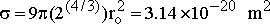4
The average total number of collisions (Nc) for a given number density (NH) is equal to: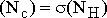5

Since the red shift (Dl¤l) per collision is b, one finds that the total red shift is equal to:6
This equation is valid for (Dl¤l) <<1.  It is therefore also valid near the solar limb, since in this case, Dl¤l»10-6
It can be seen readily that the column density of the solar hydrogen as seen from Earth varies as a secant of the angle q between the solar radius passing through the observed point and the Sun-Earth line.
One must notice that without any knowledge of the non-Doppler red shift mechanism described above, Findlay-Freundlich  proposed the same equation, since it is an excellent empirical description of the observed red shift.  Findlay-Freundlich  found that the red shift observed everywhere on the surface of the Sun (around 6100 Å) follows the equation: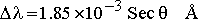7
Let us examine the coefficient (1.85´10-3 Å) measured by .  The total relative energy loss RF of these photons for q=0 are:8
Theoretically, the length of coherence of the radiation is characterized  by the Sun's surface temperature T.  From , the relative energy loss b per photon collision is given by: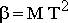9
From (2), where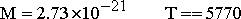K
We find: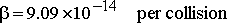10
A single photon collision on one atom undergoes a relative loss of b=9.09×10-14.  The number of collisions Nc in the Finlay-Freundlich observations  is then: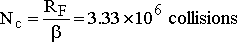11
From (4) and (5) we find:This is the column density required at q=0 degrees on the Sun to produce the observed red shift as a function of q.  From Allen [43 p. 165] we find that this column density corresponds to 277 km above the defined base of the Sun's chromosphere. Evidently, before spectral lines can be red shifted by the solar chromosphere, the atoms of iron must have produced absorption on the blackbody radiation emitted by the photosphere.  Then the solar light containing the absorption lines must travel further, through an appreciable amount of gas, so that the red shift can take place.  As expected, this calculated altitude (277 km) is just below the altitude of maximum absorption (300 km) of metals as reported , and is below a column density of 1.06 ´1022 at/cm2.  This represents then, a striking agreement with experimental data.Figure 1.
Red shift Dl of solar lines in units of 10-13 m. as a function of the position on the solar disk (for q=0 to 1.0).
The calculated CLV on the solar disk is represented in Fig. 1 as a function of Sin q and compared with the results of several other methods.  The presentation is similar to the one given by Finlay-Freundlich , (Dl=1.85´10-3 Secq   Å), can now be deduced from this recent theory ,  and from the above-calculated column density, even through the theory developed by Marmet ,  and the data reported by Allen  used here, were not originally intended for that purpose.
A second set of calculations of CLV has been performed for a different range of radii on the solar disk between 0.900<(R/Ro)<0.998, and is presented in Fig. 2.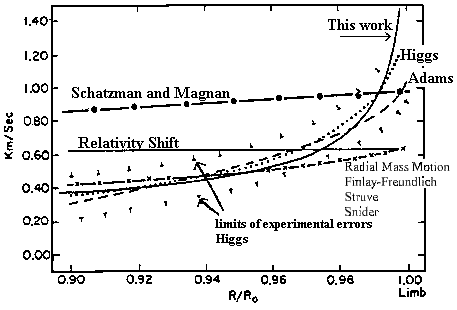Figure 2
Fig. 2. Limb effect of solar lines as a function of equivalent radial velocity.

In this case, the range of radii chosen is the same as the range of Higgs  measurements, and an error in the printed numbers on the Y axis (X2) has been corrected.  Again, the agreement with the experiments , , , and  is excellent.

IV. High-Pressure Phenomenon
A. Multi-Atom Interaction
We have seen that the red shift of spectral lines on the sun starts as expected at an altitude just below the one of maximum absorption of these lines.  This altitude (277 km) above the base of the chromosphere has also another important characteristic - it sets a threshold to a high-pressure phenomenon.  As stated previously , one can see that when the length of coherence of electromagnetic radiation is longer than the distance between atoms of the gas, one single wave train of radiation (one photon) has the possibility of interacting simultaneously on more than one atom.  In other words, one single wave train can polarize several atoms simultaneously, and the photon momentum is transferred to several electrons simultaneously.
For example, in the case of radiation interacting simultaneously on a pair of electrons (located on different atoms), the total energy emitted by bremsstrahlung by the pair of electrons that has received a given momentum is smaller than the bremsstrahlung emitted in only one electron (therefore half the mass) has received the same total momentum.  This is the consequence of the Larmor law of radiation that says that the amount of energy radiated by bremsstrahlung increases linearly with the charge accelerated, but quadratically with the acceleration given to the charge.  Consequently, it is only in the case when the gas density reaches low value, such that the length of coherence is smaller that the distance between the atoms of the gas, that one can be sure that only one atom interacts at a time and that the red shift produced follows (10).  Above that critical pressure, the red shift (per collision) becomes smaller and must practically disappear at a much higher pressure.  This means that a photon-atom interaction leads to the emission of a smaller amount of bremsstrahlung when other atoms are located within the coherence length of the interacting atom.

B. Critical Pressure
It is very difficult to determine precisely the critical pressure above which bremsstrahlung is no longer emitted a full intensity.  One can see [5, fig 1] that the time of coherence Tc of blackbody radiation at half intensity is given by the expressionThe length of coherence Lc is then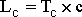Where c is the velocity of light in vacuum.  With a solar temperature T=5770 K, one finds that the length of coherence Lc is equal to 1.6737 ×10-7 m.
We have seen above that the redshift observed originates from an altitude of 277 km above the base of the chromosphere.  We know that we can detect the red shift of photons from the redshift of iron lines superimposed on the Planck`s spectrum.  There are two possible reasons why iron lines do not seem to be redshifted from an altitude lower that 277 km on the Sun:
a) It could be because there are no iron atoms at that altitude, since the absorption lines are used to detect the redshift.
b) It could be because the hydrogen pressure is too high and therefore no redshift is produced.
On the other hand, it is known ¸ that maximum iron absorption takes place at altitude 300 km, but iron atoms seem to exist at a different concentration at a lower altitude.  We can see also  that at an altitude of 277 km the gas density is 1015 at/cm3. A rough estimate of the average interatomic distance can be calculated assuming a rigid cubic geometry.  The cube root of the gas density leads to an "average" distance of AD of 10-7 m between atoms.  Since AD is not substantially smaller than the length of coherence Lc calculated above, the redshift of the iron lines observed is compatible with the calculation that the pressure effect starts to appear at about the solar altitude of 277 km.  It is not possible to determine with precision at what exact pressure the "high-pressure" phenomenon starts to appear because one cannot determine accurately the trade-off between the absence of iron atoms below 277 km and the absence of redshift due to the high-pressure phenomenon.

C. Red Shift in the Earth's Atmosphere
We have explained that electromagnetic radiation having a length of coherence much longer that the interatomic distance should not produce bremsstrahlung.  With the solar radiation the critical pressure gives about 1015 at/cm3.  This same pressure exists in the Earth's atmosphere at 73 km above the Earth's surface , and the column density above that altitude is 1.5×1019 at/cm2.  Since this is 700 times smaller than the column density above the corresponding point on the Sun (having the same density), this leads to a red shift that is 700 times smaller in the Earth's atmosphere than one observed on the Sun.  Consequently, no observable red shift is expected to be detected in the Earth's atmosphere.

V. Other Models
Let us now consider the assumption that the CLV is a consequence of a Doppler effect resulting from radial mass motion in the solar photosphere or chromosphere, as reported by Finlay-Freundlich , Struve , and Snider .  It is clear that the velocity of such a mass motion must be adjusted in order to compensate exactly for the redshift on the center of the disk, and also be adjusted exactly to the relativistic value on the limb, since any radial motion has no effect on the limb as seen from Earth.  It is easily seen in Fig 1 that between 0.5 and 0.9 of the solar disk radius, mass motion leads to a CLV in complete disagreement with experimental data.  Again, in Fig 2, above 0.900 these other models are in total disagreement with the experimental data.
Finally, let us compare the experimental results with the ones predicted using the theory of microturbulence as calculated by Schatzman and Magnan .  From [18, eq. (29) fig. 1] one can see that the red shift varies almost linearly as a function of the position on the solar radius.  The same parameters used  give a redshift on the limb of 0.35 km/s.  Furthermore, the redshift at the center of the disk is adjusted with a proper choice of the parameters E/kT. The results are shown in Fig 1 and 2, and one can notice again a serious discrepancy with the experimental data, as stated by Schatzman and Magnan  themselves: "In fact, our theory predicts too much red shifts".  Other more recent works , ,  show that both horizontal and vertical motion in the solar granulation can explain the red shift resulting from mass motion inside granules, but does not lead to the observed CLV reported by so many observers.  Furthermore, Beckers and Nelson  report: "A quantitative interpretation of the limb shift in terms of our convection model leads, however, to conclusions which are to some extent at variance with our present concept of solar granulation."
The difficulty of using the existing theories involving granules to explain CLV has been described in more detail at the beginning of this paper and can be summarized by the recent statement (1984) of Balthasar : "Up to now, no complete and satisfactory explanation for the line asymmetries known since 1956 and the limb effect known since 1907 exist."
Finally, another comment must be added since the absorption lines are relatively wide (several times wider than the redshift) and asymmetric.  Their relative displacement leading to CLV has been accurately measured, but their absolute position within the individual line profile is much more difficult to establish.  The difficulty of absolute measurements in the presence of pressure shifts and convection might account for a small constant shift across the solar disk.  In these solar measurements, the wavelength reference is the minimum  in the spectrum.  It is well known to spectroscopists that, due to line shapes and pressure broadening, precise location of atomic and molecular levels is not necessarily situated at the position of the maximum absorption, especially when the profile is asymmetric.  The accurate difference in wavelength between the actual position of an atomic level and the position of maximum absorption is still an undetermined quantity in solar lines.
At the solar disk center, it is important to notice that Balthasar  reports precise measurements showing that observed wavelengths "depends strongly on the formation depths of the lines: Lines formed in higher layers show larger redshifts."  Balthaser  also reports that lines formed in the highest layers "have shifts of about the gravitational red shift" To explain it more clearly, it is reported  that the difference between the wavelength of a given absorption line of iron observed at the disk center and formed in the highest layers of the solar chromosphere and the laboratory wavelength measurement is exactly equal to the gravitational red shift.  Furthermore, it is also suggested   that the upward velocity of "the ascending matter is decelerated". This effect explains the decreasing blueshift of the radiation issued at an increasing altitude, as observed experimentally by Crosswhite  and reported by Balthasar .  It is extremely interesting to observe that relativistic corrections, combined with the upward velocity reported , , lead to zero vertical velocity in the "highest layers".  At the solar disk center, it can also be calculated that the Doppler blueshift resulting from the upward velocity of the "lowers layers" overcompensates for the redshift produced by the gas.  We see now that the systematic investigation of the depth dependance of a lot of spectral lines done by Balthasar  was necessary for the understanding of the problem.  These conclusions are in exellent agreement with the observations of the new red shift theory presented above.

VI.  Lines Profiles
As stated previously , , experimentally measured line profiles are modified and become more asymmetric near the limb.  This asymmetry has not been clearly explained, as indicated by Higgs ,  and in more recent papers.  In the case of red shifts due to bremstrahlung on atoms, we will see that the same method used for calculating the amount of gas in the solar chromosphere can be used to determine the line profiles.  It is clear that the absorbing solar chromosphere has a non-negligible thickness, and the medium producing the absorption is the same as the one producing the red shift.  Absorption lines produced in the gas in the upper part of the solar chromosphere will not be red shifted significantly since the photons do not go through enough gas before reaching the Earth.  On the other hand, gas at a lower altitude will produce specific absorption lines that will be red shifted due to the presence of gas at a higher solar altitude before reaching the Earth.  The final result is that the absorption features will appear at slightly different wavelengths as seen from Earth, depending on the altitude or the altitude range where the absorption takes place in the solar atmosphere.  A larger altitude range will produce a broadening of the absorption lines.  Finally, if the absorption coefficient is not constant, as is the case when the FeI atoms have different temperatures and densities, the widened lines become asymmetric.  This is precisely what has been observed experimentally .
Let us assume that the line profile within a layer has a Gaussian distribution.  The absorption profile coming from the lowest layer (#1) is red shifted and added to the profile produced by the next layer (#2), and so on.  One sees that the profile from layer (#1) is more redshifted when reaching the Earth than the profile of layer #2.  A calculation using 40-Gaussian functions with linearly decreasing amplitudes has been used as an example in order to illustrate the contribution of uniformly spaced red shifts (originating from layers giving different absorption). It is found that this leads to a width three times as wide as the width of the Gaussian function (at half height).  The result of these calculations shows that the calculated profile, shown as "B" in Fig. 3, is similar to a typical asymmetric profile :A" (Fig. 3) observed by Higgs [14, fig. 4] .  For a larger column density, the calculated red shift is larger with respect to the width of the lines and has a larger asymmetry, as observed experimentally , .  Finally, it can be shown that the asymmetry can be different and even reversed if the absorption takes place in different conditions.  One must conclude that the red shift resulting from bremsstrahlung, as predicted , , leads naturally to asymmetries of the type observed in solar spectra.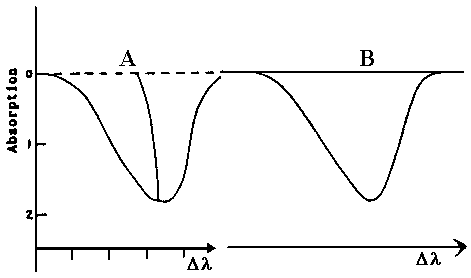Figure 3
Curve A is the line profile reported by Higgs (ref. ) (experimental)
Curve B is a typical line profile according to this work (theory)

VII.  Further Observations
Apart from the fact that the basic theory described by Marmet , explains for the first time the absolute amplitude and the shape of the CLV of spectral lines across the solar disk without and ad hoc hypothesis or adjustable parameters, several observations that have received no clear explanation previously support the theory applied here.  Let us cite two of them:
1) Very recently, Balthasar  stated: "A missing limb effect for several lines formed in very high layers was reported by Schröter *, Appenzeller and Schröter , Roca-Cortes el al. *." Such a result, which has also been observed by Snider  several years ago, is deduced naturally from the theory put forward here, since no red shift can be produced from the highest layers, because there is insufficient column density between the Sun and the Earth to produce a measurable red shift.
2) Balthasar  also reports that "a stronger limb effect for the higher formed line at 5250 Å than for the higher line at 5576 Å" has been found by Bruning .  This line-dependent variation of red shift is easy to explain with our present model.  The larger limb effect of line 5250 Å is due to the fact that the radiation has to go through a larger amount of (red shifting) gas before reaching the observer on Earth.
Thus, the theory which takes bremsstrahlung into account explains precisely the absolute amplitude of the CLV, its variation as a function of its position on the disk and near the limb, and even the change in lineshapes especially near the limb without having to introduce any ad hoc theory or parameters.

Acknowledgment
The author wished to acknowledge fruitful discussions with Dr. G. Herzberg and Dr. P. Feldman of the Herzberg Institute of Astrophysics, Prof. P. Marchand of Laval University, Dr. L. Higgs of the Division of Radio Astronomical Observatory in Pentincton, B.C. Can.,  Dr. J. W. Kierein of the Aerospace Systems Division, Dr. M. Proulx and Dr. P. Plessis of the National Research Council of Canada, Ottawa, and L. Marmet of the University of Toronto.

References
 A. L. Peratt "Dean of the Plasma Dissidents" Natural Sciences, The World and I. pp. 190-197, May 1988.
 G. Reber "Intergalactic Plasma" IEEE Trans. Plasma Sci., Vol. PS-14, pp. 678-682, Dec. 1986.
 P. Marmet, "A New Non-Doppler Redshift" département de physique, Université Laval, Québec, Canada, 1981, p. 64.
 P. Marmet, presented at the Symp. Fundamentals of Collision Processes in the Presence of Intense Laser Fields,  St. Francis Xavier University, Antigonish, N.S., Canada, Aug, 12-16, 1985.
 P. Marmet, "A New Non-Doppler Redshift"  Physics Essays, Vol. 1 pp. 24-32, 1988.
 J. Halm, Über eine bisher unbekannte verschiebung der Fraunhoferschen linien des sonnenspectrums, Astron, Nachr., Vol. 173 pp. 273-288, 1907.
 W. S. Adams, "An Investigation of the Displacements of the Spectrum Lines at the Sun's Limb",  Astrophys. J., Vol 31, pp. 30-61, 1910.
 M. G. Adam, "Interferometric Measurements of Solar wave-lengths and an Investigation of the Einstein Gravitational Displacement", Mon. Notic. Roy. Astron. Soc. Vol. 108. pp. 446-464, 1948.
 M. G. Adam, "Interferometric Measurements of Wave-lengths", Mon. Notic. Roy. Astron. Soc. Vol. 115, pp. 405-421, 1955.
 M. G. Adam, "A New Determination of the Center-to-limb change in the Solar wavelengths. "Mon. Notic. Roy. Astron. Soc. Vol. 119. pp. 460-474, 1959.
 E. Finlay-Freundlich, "Red Shift in the Spectra of Celestial Bodies", Phil. Mag. Vol. 7 pp. 303-319, 1954.
 E. H. Schröter, "Zur deutung der rotverschiebung und Mitte-Rand-variation der Fraunhoferlinien bei berücksichtigung der temperaturschwankungen der sonnenatmosphäre"  Z. Astrophys. Vol. 41, pp. 141-181, 1957.
 O. Struve, Elementary Astronomy, New York, Oxford Univ. Press. pp. 364, 1959.
 L. A. Higgs, " The solar Redshift ", Mon. Notic. Roy. Astron. Soc. Vol. 121, pp. 421-435, 1960.
 L. A. Higgs, Asymmetry of Solar Line Profiles", Mon. Notic. Roy. Astron. Soc. Vol. 124, pp. 51-59, 1962.
 R. Kh. Salman-Zade, Astron. Zh. Vol. 46. pp. 589-599, 1969. (translation: "Limb Effect of the Fraunhofer Lines in the Solar Spectrum" Sov. Astron. AJ. Vol. 13, pp. 466-472, 1969).
 J. L. Snider, "Atomic Beam Study of the 7699 Å Potassium Line and the Gravitational Red Shift" Sol. Phys. Vol. 12. pp. 352-369, 1970.
 E. Schatzman and C. Magnan, "Shifts and Asymmetries of Lines Formed in a Thermally Driven Turbulent Medium, Astron. Astrophys. Vol. 38. pp. 373-380. 1975.
 J. C. Pecker, "Décalage vers le rouge et expansion de l'univers." Paris: Éditions de CNRS, p. 619, 1977.
 J. M. Beckers and L. E. Cram, "Use of the Solar Limb Effect to test Photon Decay and Cosmological Red Shift Theories". Nature, Vol. 280, pp. 255-256, 1979.
 H. J. Reboul, "Untrivial Redshifts: A Bibliographical Catalogue". Astron. Astrophys. Suppl. Vol. 45, pp. 129-144, 1981.
 R. Howard, J. E. Boyden and B. J. Labonte, "Solar Rotation Measurements at Mount Wilson".  Sol.  Phys. Vol. 66. pp. 167-185. 1980.
 D. Dravins, "Photospheric Spectrum Line Asymmetries and Wavelengths Shifts"  Ann. Astron. Astrophys. Vol. 20, pp. 61-89, 1982.
 J. Evershed and T. Royds, "Report of the Kodaikanal Observatory", Kodaikanal Obs.  Bull. Vol. 3, pp. 145-156, 1916.
 J. L. Snider, "New Measurement of the Solar Gravitational Redshift". Phys. Rev. Lett. Vol. 28, pp. 853-856, 1972.
 F. Cavallini, G. Ceppatelli and  A. Righini, "Asymmetry and shift of Three FeI Photoscopic Lines in Solar Active Regions" Astron. Astrophys. Vol. 143, pp. 116-121, 1985.
 E. A. Gurtovenko, R. I. Kostic, T. V. Orlova, V. I. Troyan and G. L. Fedorchenko, "Profiles of Selected Fraunhofer Lines for Different Center-to-Limb Positions at the Solar Disk". Kiev, Naukova, Dunka, pp. 224, 1975.
 M. G. Adam, P. A. Ibbetson and A. D. Petford, "The Solar Limb Effect: Observation of Line Contours and Line Shifts". Mon. Notic. Roy. Astron. Soc. Vol. 177.pp. 687-708, 1976.
 P. N. Brandt and E. H. Schröter, "On the Center-to-limb Variation and Latitude Dependence  on the Asymmetry and Wavelength Shift of the Solar Line l5576."  Sol. Phys. Vol. 79. pp. 3-18. 1982.
 J. M. Beckers and G. D. Nelson, "Some Comments on the Limb Shift of Solar Lines".  Sol. Phys. Vol. 58. pp. 243-261, 1978.
 M. Kaisig, and C. J. Durrant, "The Asymmetry of Photospheric Absorption Lines". Astron. Astrophys. Vol. 116. pp. 332-340, 1982.
 J. W. Brault, "The Gravitational Red Shift in the Solar Spectrum" Ph. D. dissertation, Princeton Univ. Princeton. N. J. p. 87, 1962.
 F. Cavallini, G. Ceppatelli and A. Righini, "Meridional and Equatorial Center-to-limb Variation of the Asymmetry and shifts of Three FeI Solar Photospheric Lines around 6300 Å". Astron. Astrophys.  Preprint  7, 1985.
 S. L. Keil, "The Structure of Solar Granulation 1: Observation of the Spatial and Temporal Behavior of Vertical Motions". Astrophys. J. Vol. 237. pp. 1024-1034, 1980.
 B. J. Labonte and R. Howard, "Solar Rotation Measurements at Mount Wilson: III Meridional Flow and Limbshift"  Sol. Phys. Vol. 80, pp. 361-372, 1982.
 D. H. Bruning  "Empirical Limb Effect Curves for the FeI Lines l5250 and l5576". Sol. Phys. Vol. 71. pp. 233-236, 1981.
 I. Appenzeller and E. H. Schröter, "Center-to-limb Variations of the Intensity and the Wavelength of Several Fraunhofer Lines along the Sun's Polar and Equatorial Diameter". Astrophys. J. Vol. 147, pp. 1100-1105, 1967.
 T. L. Duvall Jr., "Large Scale Solar Velocity Fields". Ph. D. dissertation, Stanford Univ. Palo Alto CA, 1977
 T. L. Duvall Jr., "Large Scale Solar Velocity Fields".  Sol Phys. Vol. 63. pp. 3-15, 1979.
 J. W. Kierein and B. M. Sharp, Compton effect Interpretation of Solar Red Shifts", Sol. Phys. Vol. 3, pp. 450-453, 1968.
 H. A. Bethe and E. E. Salpeter, "Quantum Mechanics of One and Two Electron Atoms". Berlin Göttinger, Heidelberg: Springer-Verlag, pp. 369, 1957.
 J. M. Jauch and F. Rohrlich, "The Theory of Photons and Electrons"  New York, Berlin, Heidelberg: Springer-Verlag, p. 364, 1980.
 C. W. Allen, "Astrophysical Quantities", 3rd Edition, London: Athlone Press, Univ. of London, 1973.
 H. Balthasar, "Asymmetries and Wavelengths of Solar Spectral Lines and the Solar Rotation Determined from Fourier Transform Spectra",  Sol. Phys. Vol. 93. pp. 219-241, 1984.
 H. M. Crosswhite,  "The Iron-Neon Hollow Cathode Spectrum". J. Res. Nat. Bur. Stand. Sect. A. Vol. 79. p. 17. 1975.
 E. H. Schröter,  "Rotverschiebung und 'Limb-Effekt' im Sonnenspektrum" Mitt. Astrophys. Obs. Postdam Nr. Vol. 83. No:4, pp. 69-75, 1959
 T. Roca-Cortes, M. Vásquez, and H. Wöhl, "Space and Time Variations of K1 7699 Solar Line Profile". Sol. Phys. Vol. 88, pp. 1-8, 1983.